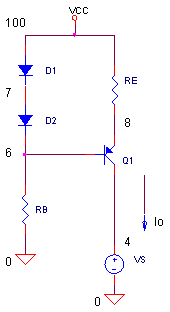eCircuit  Center AUDIO AMPLIFIER

BJT Current Source

CIRCUITOne of the less glamorous but equally important circuits in audio amplifiers is the current source. It plays two positions on the audio team: 1) bias the differential input stage and 2) provide bias AND a super high gain for the Gain Stage with its astonishingly high output resistance.  (See the Basic Audio Amplifier.)

Let's explore a few aspects of this key player. How do you set the output bias current? How well does it maintain a current as the output voltage swings? And how high is its output resistance?

BASIC CURRENT SOURCE

This circuit's claim to fame lies in providing a constant current Io, regardless of the output voltage. What determines Io? It's basically set by the voltage across RE such that VRE / RE = Ie Ic = IoTo find VRE, write a voltage loop equation around the D1, D2, Q1 and RE loop.

VD1+ VD2 -Vbe1 - VRE = 0

Now, substitute VRE Io * RE and solve for Io.

Io (VD1+ VD2 - Vbe1) / RE.

Now if VD2 Vbe1, they cancel and we can simplify the equation a bit further.

Io VD1 / RE.

Cool, a little analysis usually leads to a simpler view!. (Looking at the schematic, you might have directly deduced that VD2 approximately cancels Vbe1 leaving VD1 directly across RE.).

OUTPUT CURRENT

Let's see if this current source lives up to it's reputation. First we'll check it's output current level. Then we'll use VS to create wild voltage swings at V(4) and see if the output current remains constant.

Let's design this source for 1 mA approximately. Assuming that VD1 is about 0.7 V, set RE = VD1 / Io  = 0.7 V / 1 mA = 700 ohms. Initially set the peak of the sine wave at VS to 0V. The current source uses an MPSA56, a commonly used PNP transistor for audio applications.

CIRCUIT INSIGHT   Run a TRANSIENT ANALYSIS and plot output current IC(Q1). What is the current output? Io should read about 1 mA. It probably won't be exactly 1 mA, but not bad for a simplified equation.

HANDS-ON DESIGN   You can tweak the value of output current two ways. Try increasing RE by 10 to 20 ohms at a time. Then rerun the simulation to find the new output current. What value of RE gets you within 1% of 1mA?

As an alternative, try increasing RB by 1k at a time and rerun the simulation. How does this effect the circuit? Less current through the D1,D2 string means a smaller voltage across RE and a smaller output current.

CONSTANT CURRENT

Let's put the current source to the compliance test. How well does it maintain a constant current when the voltage is changing at V(4)? Set the peak of the sine wave at VS to 1V.

VS 4 0 AC 1 SIN(0V 1VPEAK 1KHZ)

CIRCUIT INSIGHT   Run a TRANSIENT ANALYSIS and plot output current IC(Q1). Open another plot window and plot V(4). How constant is Io as V(4) changes? You should see only a small change, maybe in the μA range of change!

Let's increase the swing at VS to 10V Peak. Rerun the simulation and check out Io. Hey, not bad! Wiith a +/-20V swing, the current only changes by a few tens of μA.

Keep increasing VS until the wheels fall off the wagon and Io no longer holds steady at 1 mA.

OUTPUT RESISTANCE

What is the output resistance of our current source? We'll find it from a simple calculation.

Ro = ΔVo / ΔIo.

Set the peak of the sine wave at VS back to 1V.

CIRCUIT INSIGHT   Run a TRANSIENT ANALYSIS plotting IC(Q1) and V(4) in separate windows. Now find the peak-to-peak change in Io and calculate Ro. A typical Ro may be

Ro = ΔVo / ΔIo
= 2 Vp-p / 3.3
μAp-p
= 600 k
Ω

This amazingly high Ro brings you kaboons of gain when placed at the collector of a common emitter amplifier. So "what's the big deal?" some would say! Just stick a 600k resistor at the collector. Yes, but then you've only got less than Vcc/Rc = 15V/600k = 25 uA current available to drive the next stage. With the current source, you've got 1 mA available to drive the next stage, while still creating 600k of load resistance. (We'll explore this in an upcoming topic.)

What happens to Ro if you increase or decrease Io by a factor of 2? Adjust RE to raise or lower Io. Did Ro increase or decrease with your change?

SPICE FILE

Download the file or copy this netlist into a text file with the *.cir extension.

BJT_CURRENT_SOURCE.CIR
*
* TEST SIGNAL
VS	4	0	AC	1 SIN(0V 0VPEAK 1KHZ)
* POWER SUPPLY
VCC	100	0	+15V
*
* BIAS DIODES
D1	100	7	DNOM
D2	7	6	DNOM
RB	6	0	15K
* BIAS RESISTOR AND PNP OUTPUT TRANSISTOR
RE	100	8	700
Q1 4 6 8 QMPSA56

* DEVICE MODELS
.MODEL DNOM D()
.MODEL Qmpsa56 pnp
+IS=9.62586e-12 BF=246.647 NF=1.19008 VAF=19.1507
+IKF=0.332189 ISE=1.74278e-11 NE=1.95504 BR=0.1
+NR=1.49124 VAR=3.92539 IKR=4.71144 ISC=3.90265e-13
+NC=3.98982 RB=0.772559 IRB=0.184627 RBM=0.365314
+RE=0.00202964 RC=0.26419 XTB=0.467199 XTI=1
+EG=0.851752 CJE=4.05433e-11 VJE=0.4 MJE=0.748564
+TF=7.64992e-10 XTF=4.80943 VTF=12.6194 ITF=0.441135
+CJC=4.21506e-11 VJC=0.4 MJC=0.352218 XCJC=0.899996
+FC=0.180025 CJS=0 VJS=0.75 MJS=0.5
+TR=1e-07 PTF=0 KF=0 AF=1
*
* ANALYSIS
*.AC DEC 5 10 100k
.TRAN 0.001MS 1MS
.PROBE
.END

Top ↑